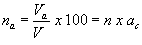# Volume Relations for Soil

As the amounts of both water and air are variable, the volume of solids is taken as the reference quantity. Thus, several relational volumetric quantities may be defined. The following are the basic volume relations:

1. Void ratio (e) is the ratio of the volume of voids (Vv) to the volume of soil solids (Vs), and is expressed as a decimal.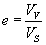2. Porosity (n) is the ratio of the volume of voids to the total volume of soil (V ), and is expressed as a percentage.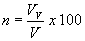Void ratio and porosity are inter-related to each other as follows: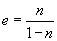and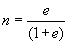3. The volume of water (Vw) in a soil can vary between zero (i.e. a dry soil) and the volume of voids. This can be expressed as the degree of saturation (S) in percentage.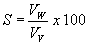For a dry soil, S = 0%, and for a fully saturated soil, S = 100%.

4. Air content (ac) is the ratio of the volume of air (Va) to the volume of voids.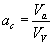5. Percentage air voids (nais the ratio of the volume of air to the total volume.